# Two-Beam Interference Equation

Excerpt from Field Guide to Interferometric Optical Testing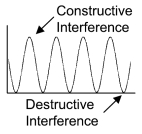Interferometric optical testing is based on the phenomena of interference. Two-beam interference is the superposition of two waves, such as the disturbance of the surface of a pond by a small rock encountering a similar pattern from a second rock. When two wave crests reach the same point simultaneously, the wave height is the sum ofthe two individual waves. Conversely, a wave trough and a wavecrest reaching a point simultaneously will cancel each other out. Water,sound, and light waves all exhibit interference, but for the purposeof optical testing, the focus will be the interference of light and its applications.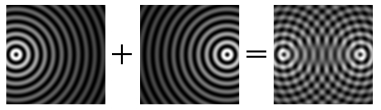A light wave can be described by its frequency, amplitude, and phase, and the resulting interference pattern between two waves depends on these properties, among others. The two-beam interference equation for monochromatic waves is: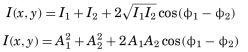• I is the irradiance. Detectors respond to irradiance, which is the electric field amplitude, A, squared: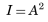• Φ is the phase of the wave in radians: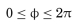• Φ1 - Φ2 = ΔΦ is the phase difference between the test and reference beams.

Citation:

E. P. Goodwin and J. C. Wyant, Field Guide to Interferometric Optical Testing, SPIE Press, Bellingham, WA (2006).